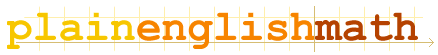About this site Fundamentals Vectors Matrices Circles and Triangles Complex Numbers Sequences and Series Calculus Appendices Glossary . . .You are here: MathematicsCircles and Triangles → Circles and Triangles

# Circles and Triangles

Have you ever picked up a scientific calculator; and wondered about the meaning of the buttons [sin], [cos] and [tan]?
Sine, Cosine and Tangent (as well as the more rarely used Secant, Cosecant and Cotangent) are actually mathematical functions; and are known as the circular functions, because they describe circles.
(They are also known as the Trigonometric functions, because they are used in trigonometry; the study of triangles.)

Don't be fooled though. The usefulness of the circular functions isn't limited to working with circles and triangles. They are also indispensible in dealing with vectors, complex numbers, calculus and wave mechanics (which deals with the ripples that affect the workings of the universe). So make sure you understand this section! But don't worry; none of this stuff is really all that hard.

## Circles

Everyone knows how to measure angles in degrees - there are 360 degrees in a circle, for instance. But what about angles in radians?

## Triangles

Pythagoras' Theorem
On right-angled triangles, and other things.

Right angled triangles
...and how to calculate their dimensions

## Circles and Triangles

sin, cos, tan...
What do these functions really represent?

## Circles and Waves

Sine waves

The Complex Exponential